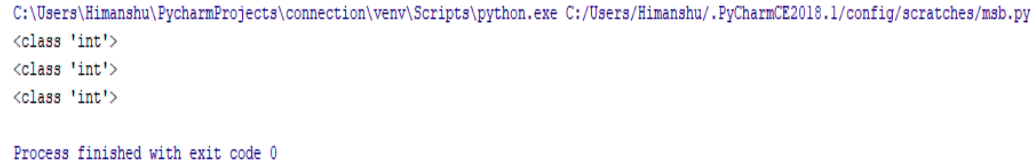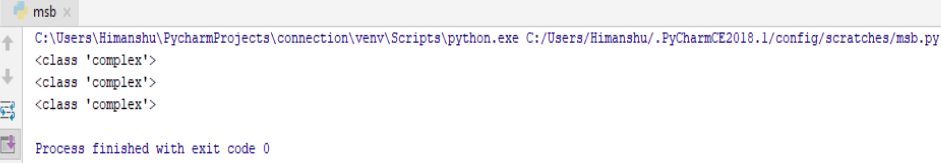×

### Html & Html5

Introduction to Html

Html5 Grid

Html5 Graphics

Html5 Media

### Python Numbers

In Python there are three type of numerics . These are as follows :-

• int
• float
• complex

### Numeric int

Integer or int includes infinite - natural numbers , whole numbers , positive or negative numbers without decimal .
So let’s see...

a=50

b=46464646746413164613215

c=-6346432886585858585

print(type(a))

print(type(b))

print(type(c))

### Output of this Program

Here in the above program, first we have assigned the different values to different variables , than through Python command we ask to Python interpreter to just tell us the type of the variable .
So here comes the output :-And it just tells us that , it is ‘int’ type variable.

### Numeric float

Float basically contains positive or negative numbers including decimals.
For example:-

a = 45.1

b=65.87

c=35.21443131464313130

print(type(a))

print(type(b))

print(type(c))

### Output of this Program

Here in the above program, first we have assigned the different decimal values to different variables , than through Python command we ask to Python interpreter to just tell us the type of the variable .
So here comes the output :-And it just tell us that it is a ‘float’ type variable.

### Numeric complex

Complex basically contains ‘j’ as the imaginary part.
For example :-

a=3+9j

b=16j

c=-24j

print(type(a))

print(type(b))

print(type(c))

### Output of this Program

Here in the above program, first we have assigned the different values to different variables including ‘j’ also which is an imaginary part , than through Python command we ask to Python interpreter to just tell us the type of the variable .
So here comes the output :-And it just tell us that it is a ‘complex’ type variable.

python training insitute| Best IT Training classes in Gurgaon | python training